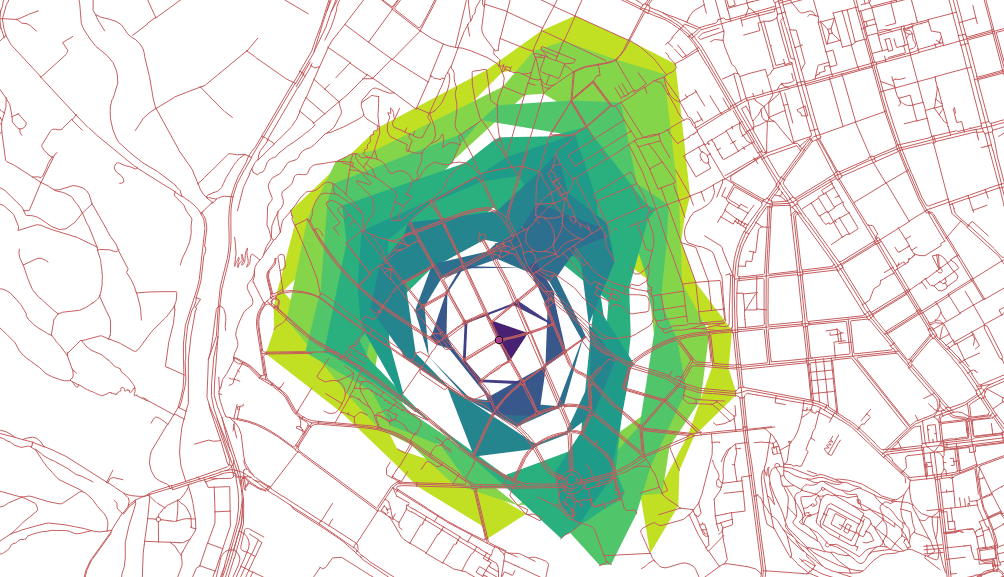## Catchment Area Calculation with pgRouting

This post is written as response to Michal Zimmermann's article "Routing with pgRouting: Catchment Area Calculation".Michal's article was interesting to read, and it definitely contains some valid points of criticism. The drive-time polygons definitely don't look as one would expect! I couldn't believe, that this is pgRouting's fault, because the result always looked OK when using the drive-time and polygon generating functions. So hwo can it be done better?

## Lack of Documentation

While drive-time (or catchment area) calculation is needed frequently, I must admit, that the related pgRouting functions are of inferior quality in the pgRouting Documentation, and they are not mentioned in the pgRouting Workshop at all. However, the use of `pgr_drivingDistance` or `pgr_pointsAsPolygon` follow the same pattern as most of the pgRouting functions. After learning through the workshop it shouldn't be too difficult to understand and use any other pgRouting function.

This article shall be the first step to provide better documentation, eventually it will be added later as a new chapter for the workshop.

## The Network is Crucial

Many problems start at the very beginning with "bad" network data. If the network data was processed with the wrong tools and doesn't contain a correct network topology, pgRouting will always return bad results. You must know very well, what you are doing, if you use a different tool than osm2pgrouting (or osm2po) with OpenStreetMap data. It's almost certain to fail, if the network topology is computed manually with different software. Its's NOT trivial!!! And using osm2pgrouting is not really difficult:

First we download some OSM data, in this case it's the same extent, defined as bounding box:

``````BBOX="16.538,49.147,16.699,49.240"
"http://www.overpass-api.de/api/xapi?*[bbox=\${BBOX}][@meta]"
``````

Then we create a database `routing` and install PostGIS and pgRouting as extension:

``````createdb routing
psql --dbname routing -c 'CREATE EXTENSION postgis'
psql --dbname routing -c 'CREATE EXTENSION pgRouting'
``````

And as the third step we use osm2pgrouting to parse and process the OSM and import it into our database. `mapconfig.xml` needs to be adjusted depending on the purpose. However in this post I'm using the default settings.

``````osm2pgrouting --f ./roads.osm \
--conf path/to/mapconfig.xml
--dbname routing
``````

The latest version of osm2pgrouting supports incremental data processing, so if your data size exceeds the required work memory, you can split the area into many and process them one by one. osm2pgrouting will make sure, that only missing network data will be added.

There is nothing else to do! The data is ready for pgRouting!

## Compute Drive Times

With `pgr_drivingDistance` we can calculate the distance (cost) from a start node to any other node in the network until a maximum cost is reached. Because our network data is rather small, the maximum cost of `3600` seconds starting from node `15632` covers the whole area. And for simplicity we store the query result in a table `distances`.

``````DROP TABLE IF EXISTS distances;
CREATE TABLE distances AS (SELECT a.node AS id, a.agg_cost AS distance, b.the_geom
FROM pgr_drivingDistance(
'SELECT gid AS id, source, target, cost_s as cost, reverse_cost_s as reverse_cost FROM public.ways',
15632,
3600,
true
) a, ways_vertices_pgr b WHERE a.node = b.id
);
``````

Note: the `cost` parameter in pgRouting can be computed as you like. `osm2pgrouting` already pre-computes some costs based on distance and on time, using the settings from the `mapconfig.xml` file.

## Generate Polygons

Now we can write a query to compute drive time polygons based on a list (array) of distances, in our case in seconds:

``````DO \$\$
DECLARE
dist int;
arr int[] := ARRAY[300,200,100,50];
BEGIN
DROP TABLE IF EXISTS catchments;
CREATE TABLE catchments(
distance integer,
the_geom geometry(polygon,4326)
);

FOREACH dist IN ARRAY arr
LOOP
RAISE INFO 'Distance is %', dist;
WITH polygon AS (
SELECT pgr_pointsAsPolygon(
'SELECT id, ST_X(the_geom) AS x, ST_Y(the_geom) AS y
FROM distances WHERE distance <= ' || dist || ';'
) AS geom
)
INSERT INTO catchments (distance,the_geom)
SELECT dist, ST_SetSRID(polygon.geom,4326) FROM polygon;
END LOOP;
END\$\$;
``````

We're computing four drive-time polygons with an upper limit of costs: 300, 200, 100 and 50 seconds. The result is written the table `catchments` and can be rendered with QGIS for example:The function `[pgr_pointsAsPolygon](9)` draws an alpha shape around a given set of points. The polygon is computed using CGAL's 2-D Alpha Shape algorithm and by default uses an optimal alpha value.

By defining a custom alpha value like `0.00001`, the fineness of the polygon can be modified:

``````DO \$\$
DECLARE
dist int;
arr int[] := ARRAY[300,200,100,50];
BEGIN
DROP TABLE IF EXISTS catchments;
CREATE TABLE catchments(
distance integer,
the_geom geometry(polygon,4326)
);

FOREACH dist IN ARRAY arr
LOOP
RAISE INFO 'Distance is %', dist;
WITH polygon AS (
SELECT pgr_pointsAsPolygon(
'SELECT id, ST_X(the_geom) AS x, ST_Y(the_geom) AS y
FROM distances WHERE distance <= ' || dist || ';',
0.00001
) AS geom
)
INSERT INTO catchments (distance,the_geom)
SELECT dist, ST_SetSRID(polygon.geom,4326) FROM polygon;
END LOOP;
END\$\$;
``````The example shows, that processing network data in a wrong manner will usually lead to wrong results. It's not always pgRouting's fault ;-)

## Remarks

• It's crucial to prepare the network data correctly!
• For the examples standard costs (seconds) were used, that do not fit for pedestrians and need some custom calibration.
• Queries can be written more elegant, for example as stored procedures to accept variables as function arguments.